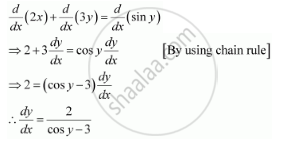Share

# Find Dy/Dx for 2x + 3y = Sin Y - CBSE (Science) Class 12 - Mathematics

ConceptDerivatives of Implicit Functions

#### Question

Find dy/dx

2x + 3y = sin y

#### Solution

The given relationship is 2x + 3y = sin y

Differentiating this relationship with respect to x, we obtainIs there an error in this question or solution?

#### APPEARS IN

NCERT Solution for Mathematics Textbook for Class 12 (2018 to Current)
Chapter 5: Continuity and Differentiability
Q: 2 | Page no. 169

#### Video TutorialsVIEW ALL 

Solution Find Dy/Dx for 2x + 3y = Sin Y Concept: Derivatives of Implicit Functions.
S